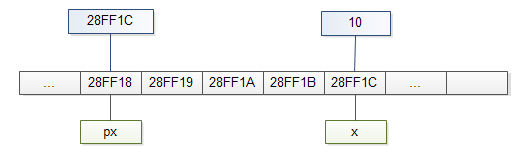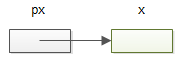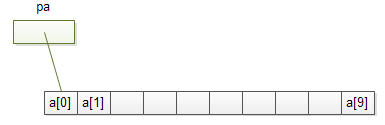# 7. C语言教程-指针

## 7. Pointer

Posted by xuepro on October 30, 2017

“教小白精通编程”系列之“C语言教程” （版权所有，不得转载，擅自抄袭转载将承担法律责任）

## 7. 指针

#include <stdio.h>

void main(){
int x = 3;
printf("x=%d,\t the address is  %d",x &x);  /*其中的&是 “取地址运算符”，用于获得一个变量的地址*/
}


类型* 变量名;


类型* 变量名 = 指针值;


#include <stdio.h>

void main(){
int x = 3;
int* px  = &x;   /*将变量i的地址用于初始化 “整型指针类型”的变量px, px 和 &x的类型都是同一个类型： “整型指针类型” */
printf("x=%d,\t the address is  %d \t %d",x &x,px);  /*其中的&是 “取地址运算符”，用于获得一个变量的地址*/
}## 通过所谓的“取内容运算符”或“间接运算符” ’*‘

   printf("%d",*px);     /* 其中的*px 就是 x */


 *px = 20;
printf("*px = %d\n",*px); /* 20 */
printf("x = %d\n",x); /* 20 */


  1)  类型*  和 类型 是两个不同的类型。比如：
int * : 也是 一个数据类型，称为整型指针类型
int : 整型


    int x = 10;
int* px = 20; /*错：两边的数据类型不一样，一个是"整型指针类型",一个是“整型” */
x = &x;  /* 同样的错误*/


  int x = 10;
int* px = 0;  /*也可以用C语言定义好的宏常量NULL来初始化： int* px = NULL;  */
/* C语言定义NULL就是0： #define NULL 0 */
px = &x;


## 指针的指针

#include <stdio.h>

void main(){
int x = 10;
int *p = &x;   /* p 保存的是x的地址，类型是“整型指针类型”*/
int ** q = &p;  /* q 保存的是p的地址，类型是“整型指针的指针类型”*/
int y = *p;
int z = *(*q);
printf("%d\t%d\t%d\t%d\t%d\n",x,&x,p,&p,q);
printf("%d\t%d\t%d\t%d\t%d\t%d\n",q,*q,**q,*p,y,z);
}


## 数组就是指针

int a;
int * pa;
pa = &a;  /*也可以写成：pa = a;
因为 a 和 &(a)都表示的是数组的起始地址
（数组的第一个元素的地址） */#include <stdio.h>

void main(){
int arr[] = {1,2,3,4,5,6,7};
int *p = arr;        /*数组名就是数组的起始地址*/
int *q = &(arr);  /*第一个元素的地址*/
printf("%d\t%d\t%d\t%d\n",arr,&(arr),p,q);
printf("%d\t%d\t%d\t%d\n",arr,*arr,*p,*q); /* 用下标运算符[]得到一个数据元素
或取内容运算符*得到整型指针指向的那个整形变量
*/
}


   /*   arr实际就是通过指针arr向后偏移2个整型量,然后通过取内容运算符*
得到偏移后的指针（arr+2）指向的那个整型量。
即： arr等价于 *(arr+2) */

#include <stdio.h>

void main(){
int arr[] = {1,2,3,4,5,6,7};
printf("%d\t%d\n",arr,*(arr+2));
*(arr+2) = 30;
printf("%d\t%d\n",arr,*(arr+2));
arr = 40;
printf("%d\t%d\n",arr,*(arr+2));
int y = arr;  /*错，下标越界了。
7个元素的数组下标只能是：
0，1，2，3，4，5，6*/
}


/*求n个元素的数组arr中的最小值*/

int Min(int arr[],int n){
int m,i;
if(n<1) return 0; /*空的数组，就假装最小值为0吧*/
m = arr;  /* 假设最小值开始为第一个元素的值*/
for(i = 1 ; i<n ;i++)
if(arr[i]<m)  /*发现更小的*/
m = arr[i];
return m;
}


/*求n个元素的数组arr中的最小值*/

int Min(int *arr,int n){
int m,i;
if(n<1) return 0; /*空的数组，就假装最小值为0吧*/
m = arr;  /* 假设最小值开始为第一个元素的值*/
for(i = 1 ; i<n ;i++)
if(arr[i]<m)  /*发现更小的*/
m = arr[i];
return m;
}


#include <stdio.h>

void main(){
int a = {34,1,56,27,8,19};
int arr = {3,19,56,7,48,23,71,2};
printf("the min of a is %d\n",Min(a,6));
printf("the min of arr is %d\n",Min(arr,8));
}


/*求n个元素的数组arr中的最小值*/

int Min(int *arr,int n){
int m,i;
if(n<1) return 0; /*空的数组，就假装最小值为0吧*/
m = arr;  /* 假设最小值开始为第一个元素的值*/
for(i = 1 ; i<n ;i++)
if( *(arr+i) < m )  /*发现更小的*/
m = *(arr+i);
return m;
}


/*求n个元素的数组arr中的最小值*/

int Min(int *arr,int n){
int *p = arr,*q = arr+n;
if(n<1) return 0; /*空的数组，就假装最小值为0吧*/
m = *p;  /* 假设最小值开始为第一个元素的值*/
for(;p<q; p++)
if( *p < m )  /*发现更小的*/
m = *p;
return m;
}


## 向函数传递数组

/*函数名param后跟一个空的[]，说明param可以接受一个数组*/
void myFunction(int param[]) {
/*函数体 代码*/
}

/*函数名param后跟一个非空的[size]，说明param可以接受一个大小为size的数组*/
void myFunction(int param[]) {
/*函数体 代码*/
}

/*函数名param前有一个*，说明param可以接受一个数组*/
void myFunction(int *param) {
/*函数体 代码*/
}


double getAverage(int arr[], int size) {

int i;
double avg;
double sum = 0;

for (i = 0; i < size; ++i) {
sum += arr[i];
}

avg = sum / size;

return avg;
}


#include <stdio.h>

/* 函数声明，表示存在这种格式的函数getAverage,

double getAverage(int arr[], int size);

int main () {

/* 5个元素的int整型数组 */
int balance = {1000, 2, 3, 17, 50};
double avg;

/* 将数组名（实际是数组对应内存块的起始地址)传给getAverage函数 */
avg = getAverage( balance, 5 ) ;

/* 输出getAverage的返回值 */
printf( "Average value is: %f ", avg );

return 0;
}


/*
* File   : main.c
* Author : zentut.com
* Purpose: Demonstrates multidimensional array & function in C
*/

#include <stdio.h>

#include <stdlib.h>

const int ROW = 2;
const int COLUMN = 3;

/*这2个函数的定义在main函数的调用语句后面，所以要先声明（说明）函数的规范*/
void fill_array(int (*pm)[COLUMN],int row);
void display(int m[][COLUMN],int row);

int main()
{
int i,j;
int m[ROW][COLUMN];

/* 修改数组元素的值 */
fill_array(m,ROW);

/* 显示数组的元素 */
display(m,ROW);

return 0;
}

void fill_array(int (*pm)[COLUMN],int row)
{

int i,j;
/* 修改数组元素的值 */
for(i = 0; i < row; i++)
{
for(j = 0; j < COLUMN; j++)
{
printf("\nm[%d][%d]:",i,j);
scanf("%d",&pm[i][j]);
}
}

}

void display(int m[][COLUMN],int row)
{
int i,j;
/* 显示数组的元素 */
printf("Array's content:\n");

for(i = 0; i < row; i++)
{
for(j = 0; j < COLUMN; j++)
{
printf("%d\t",m[i][j]);
}
printf("\n");
}

}


Please fill the array's content:

m:1

m:2

m:3

m:4

m:5

m:6
Array's content:
1       2       3
4       5       6


## 练习